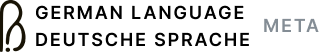Approximate recalculation of user reputation

0

(click on this box to dismiss)Q&A about the site for speakers of German wanting to discuss the finer points of the language and translation

-- Calculates an approximate of user reputation
-- takes into account:
--  * accepts
--  * bounties
--  * community wiki posts

DECLARE @UserID int = ##UserId##;
DECLARE @DailyCap int = 200;

create table #VotesByDay (Beneficiary int, CDay int, Amount int);

SELECT
v.Id as Id,
p.OwnerUserId as Beneficiary,
(CASE v.VoteTypeId + 32*(p.PostTypeId + 32*(CASE WHEN v.CreationDate >= p.CommunityOwnedDate THEN 1 ELSE 0 END))
WHEN 2+32*1 THEN 5   -- Question UP
WHEN 2+32*2 THEN 10  -- Answer UP
WHEN 3+32*1 THEN 0   -- Question DOWN
WHEN 3+32*2 THEN -2  -- Answer DOWN
ELSE 0
END) as Amount,
v.CreationDate as CreationDate
FROM
LEFT JOIN Posts p on v.PostId = p.Id
WHERE
(v.VoteTypeId = 2 or v.VoteTypeId = 3)),
SELECT v.Beneficiary, CONVERT(int, v.CreationDate) as CDay,
(CASE WHEN COALESCE(SUM(v.Amount),0) >= @DailyCap THEN @DailyCap
ELSE COALESCE(SUM(v.Amount),0) END) as Capped
FROM
GROUP BY
v.Beneficiary, CONVERT(int, v.CreationDate)),
SELECT
p.OwnerUserId as Beneficiary,
CONVERT(int, v.CreationDate) as CDay,
SUM (CASE
WHEN v.VoteTypeId = 2 OR v.VoteTypeId = 3 THEN 0
WHEN v.VoteTypeId = 1 THEN 15  -- Answer Accept
WHEN v.VoteTypeId = 8 THEN COALESCE(-v.BountyAmount,0)
WHEN v.VoteTypeId = 9 THEN COALESCE(v.BountyAmount,0)
ELSE 0
END) as Capped
FROM
INNER JOIN Posts p on v.PostId = p.Id
GROUP BY
p.OwnerUserId, CONVERT(int, v.CreationDate))

SELECT TOP 20
u.DisplayName,
u.Id,
u.Reputation,
SUM(r.Amount) as Recalculated,
u.Reputation - SUM(r.Amount) as DiffHold tight while we fetch your results